Next: Other special cases. Up: Building additional iterators Previous: Substitute all non-polynomials.

#### Transcendental Iterators.

The technique of explicitly finding roots of polynomials can be extended to cases involving transcendental functions with multivalued inverse functions.

Consider the equation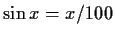. Providing for all solutions of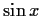yields three iterators: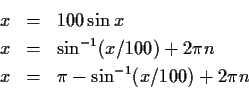where n iterates over all of the integers and the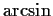returns the principal branch. The first iterator diverges chaotically. The other two, however, either converge to solutions or else fail due to theevaluating to a complex number ( |x/100| > 1).

Furthermore, all solutions can be generated by simply using different values of n. For the first equation, values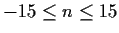will yield the even numbered roots, whereas values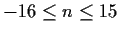will yield the remaining roots when used in the second equation. Other values of n diverge. (The asymmetry comes from the positive sign on the first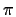; the iterator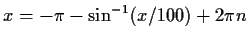would supply the same set of roots with). The solution obtained is unrelated to the initial guess used; almost any starting value acceptable to theconverges to the same root for a given choice of iterator and n.

Gaston Gonnet
1998-07-08